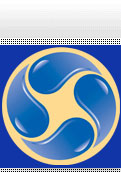# Trigonometry Tutoring

Trigonometry (from Greek trigo-non "triangle" + metron "measure")is a branch of mathematics that deals with triangles, particularly those plane triangles in which one angle has 90 degrees (right triangles). Trigonometry deals with relationships between the sides and the angles of triangles and with the trigonometric functions, which describe those relationships. Trigonometry has applications in both pure mathematics and in applied mathematics, where it is essential in many branches of science and technology. It is usually taught in secondary schools either as a separate course or as part of a precalculus course. Trigonometry is informally called "trig". A branch of trigonometry, called spherical trigonometry, studies triangles on spheres, and is important in astronomy and navigation.

 Phone: (866) 414 2030 Toll Free (310) 405 0721 (818) 850 2030 Fax: (866) 669 9584 Email: tutor@tutoringinla.com